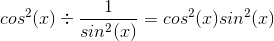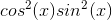Precalculus : Use Pythagorean Identities

Example Questions

Example Question #5 : Fundamental Trigonometric Identities

Simplify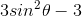.Explanation:

Write the Pythagorean Identity.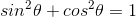Reorganize the left side of this equation so that it matches the form:Subtract cosine squared theta on both sides.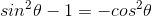Multiply both sides by 3.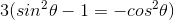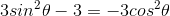Example Question #6 : Fundamental Trigonometric Identities

Which of the following is equivalent to the following expression?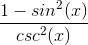Explanation:

Which of the following is equivalent to the following expression?Recall our Pythagorean trig identity: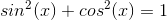It can be rearranged to look just like our numerator: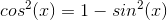So go ahead and change our original expression to: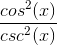Then recall the definition of cosecant: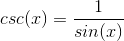So our original expression can be rewritten as: